# Acute Triangle - Definition with Examples

The Complete K-5 Math Learning Program Built for Your Child

• 30 Million Kids

Loved by kids and parent worldwide

• 50,000 Schools

Trusted by teachers across schools

• Comprehensive Curriculum

Aligned to Common Core

What is Acute Triangle?

An acute-angled triangle is a type of triangle in which all the three internal angles of the triangle are acute, that is, they measure less than 90°.

Here are some examples of acute triangles.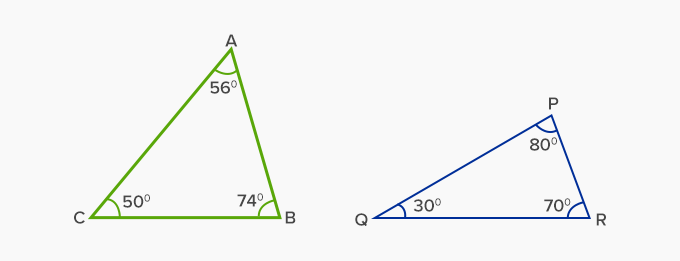Types of Acute Triangles:

There are broadly three types of acute triangles:

 Equilateral Acute Triangle: Isosceles Acute Triangle: Scalene Acute Triangle: All the interior angles of an equilateral acute triangle measure 60°. It is also known as equiangular triangle.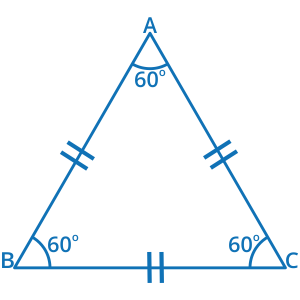Two angles of an isosceles acute triangle that measure the same, just  like its two sides.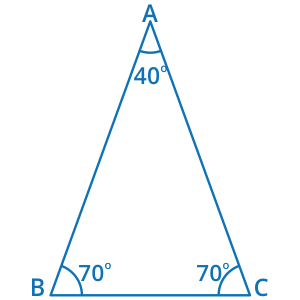All three sides and internal angles of a scalene acute triangle are unequal, that is, all angles measure less than 90 degrees.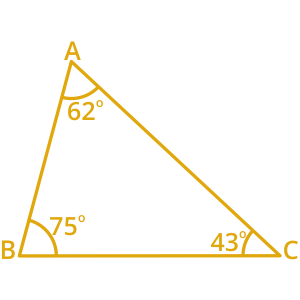Area of an acute triangle:

Area of a triangle = 12 Base X Height

Here AD is the height, and BC is the base. So, the area can be calculated by simply putting in the values in the above formula.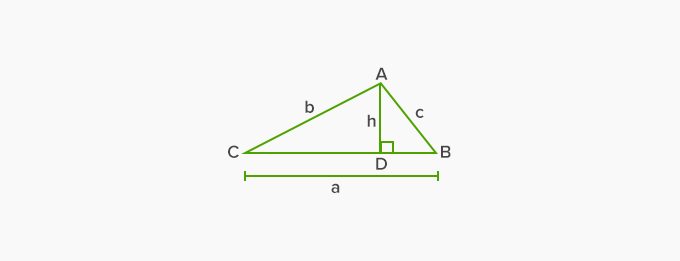Fun Facts about Acute Triangles: The angles of an acute triangle add up to 180°, because of the Angle Sum Property. A triangle cannot be acute-angled and right-angled at the same time. A triangle cannot be obtuse-angled and acute-angled simultaneously.

Acute Triangles in Nature:

Acute triangles can be seen in a variety of forms. Here are some real life examples of acute triangles:Won Numerous Awards & Honors